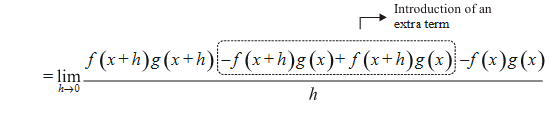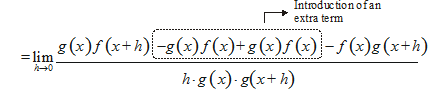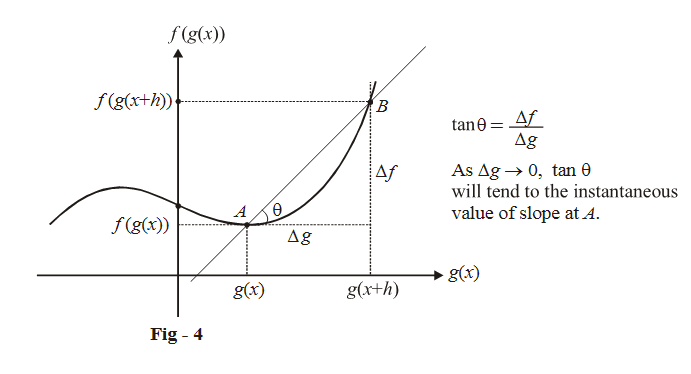# Rules Of Differentiation

Go back to  'LCD'

## RULES OF DIFFERENTIATION

Now, we will see certain general rules pertaining to differentiation that will help us in calculating the derivative of an arbitrary function without using first principles.

In the discussion that follows, we assume that $$f\left( x \right){\rm{and}}\,g\left( x \right)$$ are two differentiable functions

Rule 1: $$\boxed{\frac{{d\left( {kf\left( x \right)} \right)}}{{dx}} = \frac{{kd\left( {f\left( x \right)} \right)}}{{dx}}}$$

This rule says that a constant can be taken out from the argument of the differentiation operator. The proof is very straight forward:

$\frac{{d\left( {kf\left( x \right)} \right)}}{{dx}} = \mathop {\lim }\limits_{h \to 0} \frac{{kf\left( {x + h} \right) - kf\left( x \right)}}{h}$

\begin{align}& = k\mathop {\lim }\limits_{h \to 0} \frac{{f\left( {x + h} \right) - f\left( x \right)}}{h}\\ &= \frac{{kd\left( {f\left( x \right)} \right)}}{{dx}}\end{align}

Rule 2: $$\boxed{\frac{{d\left( {f\left( x \right) \pm g\left( x \right)} \right)}}{{dx}} = \frac{{d\left( {f\left( x \right)} \right)}}{{dx}} \pm \frac{{d\left( {g\left( x \right)} \right)}}{{dx}}}$$

This rule says that the differentiation operator is distributive over addition and subtraction. The proof for this is again quite straightforward:

\begin{align}&\frac{{d\left( {f\left( x \right) \pm g\left( x \right)} \right)}}{{dx}} = \mathop {\lim }\limits_{h \to 0} \frac{{\left\{ {f\left( {x + h} \right) \pm g\left( {x + h} \right)} \right\} - \left\{ {f\left( x \right) \pm g\left( x \right)} \right\}}}{h}\\ \end{align}

\begin{align}& = \mathop {\lim }\limits_{h \to 0} \frac{{\left\{ {f\left( {x + h} \right) - f\left( x \right)} \right\} \pm \left\{ {g\left( {x + h} \right) - g\left( x \right)} \right\}}}{h}\\ &= \mathop {\lim }\limits_{h \to 0} \frac{{f\left( {x + h} \right) - f\left( x \right)}}{h} \pm \mathop {\lim }\limits_{h \to 0} \frac{{g\left( {x + h} \right) - g\left( x \right)}}{h}\\ &= \frac{{d\left( {f\left( x \right)} \right)}}{{dx}} \pm \frac{{d\left( {g\left( x \right)} \right)}}{{dx}}\end{align}

Rule 3: $$\boxed{\frac{{d\left\{ {f\left( x \right)g\left( x \right)} \right\}}}{{dx}} = f\left( x \right)\frac{{d\left( {g\left( x \right)} \right)}}{{dx}} + g\left( x \right)\frac{{d\left( {f\left( x \right)} \right)}}{{dx}}}$$

This rule, called the Product rule, is of great help in evaluating the derivative of the product of two (or more) functions.

The proof is as follows:

\begin{align}\frac{{d\left\{ {f\left( x \right)g\left( x \right)} \right\}}}{{dx}} = \mathop {\lim }\limits_{h \to 0} \frac{{f\left( {x + h} \right)g\left( {x + h} \right) - f\left( x \right)g\left( x \right)}}{h}\end{align}\begin{align} &= \mathop {\lim }\limits_{h \to 0} \frac{{f\left( {x + h} \right)\left\{ {g\left( {x + h} \right) - g\left( x \right)} \right\} + g\left( x \right)\left\{ {f\left( {x + h} \right) - f\left( x \right)} \right\}}}{h}\\& = \mathop {\lim }\limits_{h \to 0} f\left( {x + h} \right)\mathop {\lim }\limits_{h \to 0} \frac{{g\left( {x + h} \right) - g\left( x \right)}}{h} + g\left( x \right)\mathop {\lim }\limits_{h \to 0} \frac{{f\left( {x + h} \right) - f\left( x \right)}}{h}\\&= f\left( x \right)\frac{{d\left( {g\left( x \right)} \right)}}{{dx}} + g\left( x \right)\frac{{d\left( {f\left( x \right)} \right)}}{{dx}}\end{align}

Rule 4: $$\boxed{\frac{d}{{dx}}\left\{ {\frac{{f\left( x \right)}}{{g\left( x \right)}}} \right\} = \frac{{g\left( x \right)\frac{{d\left( {f\left( x \right)} \right)}}{{dx}} - f\left( x \right)\frac{{d\left( {g\left( x \right)} \right)}}{{dx}}}}{{{{\left( {g\left( x \right)} \right)}^2}}};{\text{wherever }}g\left( x \right) \ne 0}$$

This rule, called the Quotient rule, helps us evaluate the derivative of the ratio of two functions $$f\left( x \right)/g\left( x \right),$$ wherever $$g\left( x \right) \ne 0$$ .

\begin{align}\frac{d}{{dx}}\left\{ {\frac{{f\left( x \right)}}{{g\left( x \right)}}} \right\} = \mathop {\lim }\limits_{h \to 0} \frac{{\frac{{f\left( {x + h} \right)}}{{g\left( {x + h} \right)}} - \frac{{f\left( x \right)}}{{g\left( x \right)}}}}{h}\end{align}\begin{align}&= \mathop {\lim }\limits_{h \to 0} \frac{1}{{g\left( x \right) \cdot g\left( {x + h} \right)}}\mathop {\lim }\limits_{h \to 0} \left[ {\frac{{g\left( x \right)\left\{ {f\left( {x + h} \right) - f\left( x \right)} \right\}}}{h} + f\left( x \right)\frac{{\left\{ {g\left( x \right) - g\left( {x + h} \right)} \right\}}}{h}} \right]\\ &= \frac{1}{{{{\left( {g\left( x \right)} \right)}^2}}} \cdot \left[ {g\left( x \right)\frac{{d\left( {f\left( x \right)} \right)}}{{dx}} - f\left( x \right)\frac{{d\left( {g\left( x \right)} \right)}}{{dx}}} \right]\\ & = \frac{{g\left( x \right)f'\left( x \right) - f\left( x \right)g'\left( x \right)}}{{{{\left( {g\left( x \right)} \right)}^2}}}\end{align}

Rule 5: $$\boxed{\frac{{d\,f\left( {g\left( x \right)} \right)}}{{dx}} = \frac{{d\,f\left( {g\left( x \right)} \right)}}{{d\left( {g\left( x \right)} \right)}} \cdot \frac{{d\left( {g\left( x \right)} \right)}}{{dx}}}$$

This can be stated more conveniently as

$${\left( {f\left( {g\left( x \right)} \right)} \right)^\prime} = f^\prime\left( {g\left( x \right)} \right)g^\prime\left( x \right)$$

This rule, called the Chain rule, is extremely useful to differentiate composite functions, and will be used extensively. It says that to differentiate $$f\left( {g\left( x \right)} \right),$$ we first differentiate f with respect to $$g\left( x \right)$$ and not x(i.e, we treat g(x) as a variable y and differentiate f with respect to y) and then we multiply this by the derivative of $$g\left( x \right)\left( {{\rm{or}}\,y} \right)$$ with respect to x.

\begin{align}\frac{{d\left[ {f\left( {g\left( x \right)} \right)} \right]}}{{dx}} &= \mathop {\lim }\limits_{h \to 0} \frac{{f\left( {g\left( {x + h} \right)} \right) - f\left( {g\left( x \right)} \right)}}{h}\\&= \mathop {\lim }\limits_{h \to 0} \frac{{f\left( {g\left( {x + h} \right)} \right) - f\left( {g\left( x \right)} \right)}}{{g\left( {x + h} \right) - g\left( x \right)}} \cdot \frac{{g\left( {x + h} \right) - g\left( x \right)}}{h}\end{align}

Notice carefully that the first ratio in the limit above is actually the derivative of f, but with respect to $$g\left( x \right)$$ as the variable $$\left\{ {{\rm{as}}\,h \to 0,\,\left( {g\left( {x + h} \right) - g\left( x \right)} \right) \to 0} \right\}$$ .

You can view this graphically as follows:Therefore,

\begin{align}\frac{{d\left[ {f\left( {g\left( x \right)} \right)} \right]}}{{dx}} = \frac{{d\,f\left( {g\left( x \right)} \right)}}{{d\left( {g\left( x \right)} \right)}} \cdot \frac{{d\left( {g\left( x \right)} \right)}}{{dx}}\end{align}

$$= f'\left( {g\left( x \right)} \right) \cdot g'\left( x \right)$$

For example,

$$\frac{{d\left( {\sin \left( {{x^2}} \right)} \right)}}{{dx}} =$$ $$\underbrace{\cos \left( {{x}^{2}} \right)}_{\overset{\uparrow }{\mathop{\begin{smallmatrix} \text{Derivative of} \\ \sin ({{x}^{2}})w.r.t({{x}^{2}}) \end{smallmatrix}}}\,}\cdot \underbrace{2x}_{\overset{\uparrow }{\mathop{\begin{smallmatrix} \text{Derivative of} \\ ({{x}^{2}})w.r.tx \end{smallmatrix}}}\,}$$

$$\frac{d\left( \log \left( \sin x \right) \right)}{dx}=\underbrace{\frac{1}{\sin x}}_{\begin{matrix} \downarrow \\ \begin{smallmatrix} \text{Derivative of}\log \\ (\sin x)w.r.t\,\,\sin x \end{smallmatrix} \\\end{matrix}}\cdot \underbrace{\cos x}_{\begin{matrix} \downarrow \\ \begin{smallmatrix} \text{Derivative of} \\ \sin x\,\,w.r.t\,x \end{smallmatrix} \\\end{matrix}}$$

and so on. We will encounter lots examples of the application of this rule soon.

You must note here that for this rule to be applicable at any x = a the function f(x) must be differentiable at x = g(a), since the variable that is the argument (input) to f is g(x){and not x}.